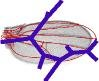Klingenberg lab FindSteinerTreeFindSteinerTree is a program for computing Steiner Trees in multiple dimensions, written by Christian Peter Klingenberg. It is an implementation of the algorithm described by Warren D. Smith (Smith, W. D. 1992. How to find Steiner minimal trees in Euclidean d-space. Algorithmica 7:137–177). This algorithm uses a branch-and-bound approach. It will therefore find the optimal tree, but the computational effort increases very rapidly with increasing numbers of data points. Smith's algorithm uses Euclidean distance as the minimizing criterion. It therefore computes the tree that connects the data points entered with tree that has the shortest possible sum of branch lengths measured as Euclidean distance (Euclidean Steiner tree). In addition to this, the program also can use the sum of squared branch lengths as the criterion (Squared-change Steiner tree). This is an extension of Smith's algorithm useful, for example, in phylogenetic studies where squared-change parsimony is much more widely used than the Euclidean criterion. Users can choose one of these options on the opening screen of the program. The program expects a text file with the input data in the following format (this example is for the corners of a cube): 8 3 0 0 0 1 0 0 0 1 0 0 0 1 0 1 1 1 0 1 1 1 0 1 1 1 The first line has two entries: the number of data points and the number of dimensions. The subsequent lines are the coordinates of the data points. (The example is a cube in three dimensions, and is available for download as the file cube 3D.txt.) There must be at least three data points. There is no upper limit to the number of data points, but performance is expected to become very slow with large numbers of points. There is no limitation of the number of dimensions (but there must be at least 2 dimensions). The coordinates of each point are entered as a line of numbers with spaces or tabs between them. Each line must have as many entries as the number of dimensions indicated in the first line of the file. The output file provides the following information: New minimum length: 6.231823467204391 New minimum length: 6.228036320023694 New minimum length: 6.1961524227066915 New minimum length: 6.196152422706691 New minimum length: 6.196152422706687 Trees examined: 1069 Topology-describing vector: 2 4 4 2 6 Steiner points: number, coordinates 9 0.24999999956831492 0.4999999990224896 0.14433756763500313 10 0.499999998802091 0.49999999891952684 0.28867513507686016 11 0.49999999906343157 0.499999998618031 0.7113248649231403 12 0.499999999152681 0.24999999959763286 0.8556624322949369 13 0.7500000008133021 0.4999999990224895 0.14433756672306342 14 0.49999999915268095 0.7500000008426198 0.8556624333469969 Edges: 1 9 2 13 3 9 4 12 9 10 5 14 10 11 6 12 11 12 7 13 10 13 8 14 11 14 Done! The first block of entries lists the successive optimal values for the total tree length. The last of these values is the length of the minimal Steiner tree. Depending on the user's choice, this is either Euclidean distance or squared Euclidean distance. This block also gives the number of trees examined. The second block is the topology-describing vector. This is further explained in the article by Smith. The third block contains the Steiner points (the internal nodes of the Steiner tree). In each line, there is the number of the respective point and its coordinates. The numbers start at the number of points in the input data plus one. The final piece of information is the edges of the tree. Each line describes the connections between the data points and Steiner points. For instance, data point 1 is connected to Steiner point 9 (first line), and Steiner point 9 is in turn connected to Steiner point 10 (5th line)...   The software is written in Java, and includes external libraries that are also written in Java. As a result, the program should be platform-independent. All what you will need to run it is a recent Java Runtime Environment (minimum Version 1.4.2) for your operating system, which is available for free from http://www.java.com. For Windows operating systems, you can download FindSteinerTree-install.jar. This is an executable .jar file that contains an installer, which will set up FindSteinerTree on your system to behave like an ordinary Windows application. But as mentioned above, you do need a Java Runtime Environment to run the installer and the program itself. For all other operating systems, download the zipped file FindSteinerTree.zip, which can be expanded into the folder named FindSteinerTree, which contains the resources needed to run the program. save it to disk and use the method appropriate for your operating system and Java Runtime Environment to start the executable .jar file FindSteinerTree.jar.